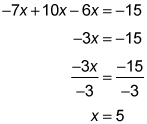##### Basic Math and Pre-Algebra Workbook For Dummies, with Online Practice, 3rd EditionWhen an algebraic equation contains fractions, you can use cross-multiplication to solve the equation. The following practice questions contain two equal fractions, where you need to cross-multiply to solve them.

## Practice questions

1. Rearrange the equation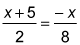to solve for x.

2. Solve the equation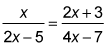1. x = –4

Remove the fraction from the equation by cross-multiplying: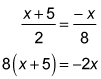Multiply to remove the parentheses from the left side of the equation:

8x + 40 = –2x

At this point, you can solve for x: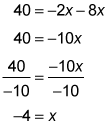2. x = 5

Remove the fractions from the equation by cross-multiplying: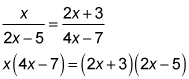Remove the parentheses from the left side of the equation by multiplying through by x; remove parentheses from the right side of the equation by FOILing:

4x2 – 7x = 4x2 – 10x + 6x – 15

Rearrange the equation:

4x2 – 7x – 4x2 + 10x – 6x = –15

Notice that the two x2 terms cancel each other out: